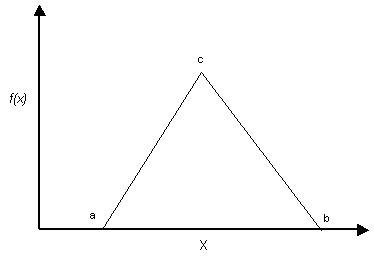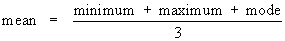# Triangular Distribution

You may wish to use a Triangular distribution in some cases, as a rough approximation to a random variable with an unknown distribution.

A Triangular distribution is specified by its minimum, maximum and mean values. It does not have to be symmetric, it can be skewed to the left or right by entering a mean value less than or greater than the average of the minimum and maximum values.

Triangular probability density function. Minimum = a, maximum = b, mode = c. For a symmetric distribution, mean = modeNOTE: For a non-symmetric Triangular distribution, the mean value is not equal to the mode. The mode is the value of the variable at the peak of the Triangular distribution. In general for a Triangular distribution, the mean is given by:• If the distribution is symmetric, then the mean is equal to the mode.
• For a left triangular distribution, the mode = minimum, and the mean = (2*minimum + maximum) / 3.
• For a right triangular distribution, the mode = maximum, and the mean = (2*maximum + minimum) / 3.